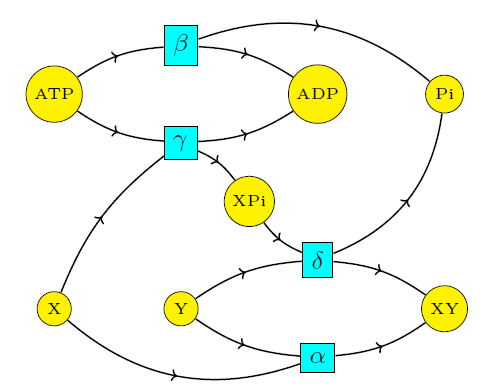## Coupling Through Emergent Conservation Laws (Part 4)

#### John Baez, Jonathan Lorand, Blake Pollard, and Maru Sarazola

We've been trying to understand coupling: how a chemical reaction that 'wants to happen' because it decreases the amount of free energy can drive forward a chemical reaction that increases free energy.

For coupling to occur, the reactant species in both reactions must interact in some way. Indeed, in real-world examples where ATP hydrolysis is coupled to the formation of larger molecule $$\mathrm{XY}$$ from parts $$\mathrm{X}$$ and $$\mathrm{Y},$$ it is observed that, aside from the reactions we discussed last time:

$$\begin{array}{cclc} \mathrm{X} + \mathrm{Y} & \mathrel{\substack{\alpha_{\rightarrow} \\\longleftrightarrow\\ \alpha_{\leftarrow}}} & \mathrm{XY} & \qquad (1) \\ \\ \mathrm{ATP} & \mathrel{\substack{\beta_{\rightarrow} \\\longleftrightarrow\\ \beta_{\leftarrow}}} & \mathrm{ADP} + \mathrm{P}_{\mathrm{i}} & \qquad (2) \end{array}$$

two other reactions (and their reverses) take place:

$$\begin{array}{cclc} \mathrm{X} + \mathrm{ATP} & \mathrel{\substack{\gamma_{\rightarrow} \\\longleftrightarrow\\ \gamma_{\leftarrow}}} & \mathrm{ADP} + \mathrm{XP}_{\mathrm{i}} & (3) \\ \\ \mathrm{XP}_{\mathrm{i}} +\mathrm{Y} & \mathrel{\substack{\delta_{\rightarrow} \\\longleftrightarrow\\ \delta_{\leftarrow}}} & \mathrm{XY} + \mathrm{P}_{\mathrm{i}} & (4) \end{array}$$

We can picture all four reactions (1-4) in a single Petri net as follows:Taking into account this more complicated set of reactions, which are interacting with each other, is still not enough to explain the phenomenon of coupling. To see this, let's consider the rate equation for the system comprised of all four reactions. To write it down neatly, let's introduce reaction velocities that say the rate at which each forward reaction is taking place, minus the rate of the reverse reaction:

$$\begin{array}{ccl} J_\alpha &=& \alpha_\to [\mathrm{X}][\mathrm{Y}] - \alpha_\leftarrow [\mathrm{XY}] \\ \\ J_\beta &=& \beta_\to [\mathrm{ATP}] - \beta_\leftarrow [\mathrm{ADP}] [\mathrm{P}_{\mathrm{i}}] \\ \\ J_\gamma &=& \gamma_\to [\mathrm{ATP}] [\mathrm{X}] - \gamma_\leftarrow [\mathrm{ADP}] [\mathrm{XP}_{\mathrm{i}} ] \\ \\ J_\delta &=& \delta_\to [\mathrm{XP}_{\mathrm{i}} ] [\mathrm{Y}] - \delta_\leftarrow [\mathrm{XY}] [\mathrm{P}_{\mathrm{i}}] \end{array}$$

All these follow from the law of mass action, which we explained in Part 2. Remember, this says that any reaction occurs at a rate equal to its rate constant times the product of the concentrations of the species involved. So, for example, this reaction

$$\mathrm{XP}_{\mathrm{i}} +\mathrm{Y} \mathrel{\substack{\delta_{\rightarrow} \\\longleftrightarrow\\ \delta_{\leftarrow}}} \mathrm{XY} + \mathrm{P}_{\mathrm{i}}$$

goes forward at a rate equal to $$\delta_\rightarrow [\mathrm{XP}_{\mathrm{i}}][\mathrm{Y}]$$, while the reverse reaction occurs at a rate equal to $$\delta_\leftarrow [\mathrm{ADP}] [\mathrm{P}_{\mathrm{i}}]$$. So, its reaction velocity is

$$J_\delta = \delta_\to [\mathrm{XP}_{\mathrm{i}} ] [\mathrm{Y}] - \delta_\leftarrow [\mathrm{XY}] [\mathrm{P}_{\mathrm{i}}]$$

In terms of these reaction velocities, we can write the rate equation as follows:

$$\begin{array}{ccl} \dot{[\mathrm{X}]} & = & -J_\alpha - J_\gamma \\ \\ \dot{[\mathrm{Y}]} & = & -J_\alpha - J_\delta \\ \\ \dot{[\mathrm{XY}]} & = & J_\alpha + J_\delta \\ \\ \dot{[\mathrm{ATP}]} & = & -J_\beta - J_\gamma \\ \\ \dot{[\mathrm{ADP}]} & = & J_\beta + J_\gamma \\ \\ \dot{[\mathrm{P}_{\mathrm{i}}]} & = & J_\beta + J_\delta \\ \\ \dot{[\mathrm{XP}_{\mathrm{i}} ]} & = & J_\gamma -J_\delta \end{array}$$

This makes sense if you think a bit: it says how each reaction contributes to the formation or destruction of each species.

In a steady state, all these time derivatives are zero, so we must have

$$J_\alpha = J_\beta = -J_\gamma = - J_\delta$$

Furthermore, in a detailed balanced equilibrium, every reaction occurs at the same rate as its reverse reaction, so all four reaction velocities vanish! In thermodynamics, a system that's truly in equilibrium obeys this sort of detailed balance condition.

When all the reaction velocities vanish, we have:

$$\begin{array}{ccl} \displaystyle{ \frac{[\mathrm{XY}]}{[\mathrm{X}][\mathrm{Y}]} } &=& \displaystyle{ \frac{\alpha_\to}{\alpha_\leftarrow} } \\ \\ \displaystyle{ \frac{[\mathrm{ADP}][\mathrm{P}_{\mathrm{i}}]}{[\mathrm{ATP}]} } &=& \displaystyle{ \frac{\beta_\to}{\beta_\leftarrow} } \\ \\ \displaystyle{ \frac{[\mathrm{ADP}] [\mathrm{XP}_{\mathrm{i}} ]}{[\mathrm{ATP}][\mathrm{X}]} } &=& \displaystyle{ \frac{\gamma_\to}{\gamma_\leftarrow} } \\ \\ \displaystyle{ \frac{[\mathrm{XY}][\mathrm{P}_{\mathrm{i}}]}{[\mathrm{XP}_{\mathrm{i}} ][\mathrm{Y}]} } &=& \displaystyle{ \frac{\delta_\to}{\delta_\leftarrow} } \end{array}$$

Thus, even when the reactants interact, there can be no coupling if the whole system is in equilibrium, since then the ratio $$[\mathrm{XY}]/[\mathrm{X}][\mathrm{Y}]$$ is still forced to be $$\alpha_\to/\alpha_\leftarrow$$. This is obvious to anyone who truly understands what Boltzmann and Gibbs did. But here we saw it in detail.

The moral is that coupling cannot occur in equilibrium. But how, precisely, does coupling occur? Stay tuned!